# 1. Multiscalar Territorial Analysis for Policy Study

The aim of this case-study consists in exploring functions proposed by the `MTA` package in a structured path of investigation (following the logic proposed by HyperAtlas) by associating MTA functions with relevant maps and plots.
In this vignette, we will investigate the concrete example of income inequalities in the Metropolis of Greater Paris (Métropole du Grand Paris).
Several elements must be considered: the study area, the territorial hierarchy and the selected indicator.

• Metropolis of Greater Paris (MGP) : a study area making sense in a political context.
The MGP has been created the 1st January 2016 after a prefiguration mission and aims at finding solutions to improve and develop the potential of the Paris metropolitan area to be more present at international level (following the initiatives that took place in London, New-York or Tokyo). The official website (last visit: 2019-10) of this infrastructure provides all the details of this new level of governance. Namely, this spatial planning project proposes to “develop a stronger solidarity between territories, reduce territorial inequalities and propose a reequilibrium in term of access to household, employment, training, services and amenities”. For this emerging zoning of spatial planning, the need for indicators and analysis of territorial inequalities is high. The MTA methodology seems to be particularly adapted to propose territorial evidences in this context.

• From the IRIS to the MGP, a lot of hierarchical territorial divisions are available.
The lowest territorial level existing in France associated with public statistical information is IRIS (Ilots Regroupés pour l'Information Statistique). The reference size targeted for each IRIS is 2000 inhabitants (last visit: 2019-10). Each municipality over 10 000 inhabitants and a high proportion of municipalities between 5 000 and 10 000 inhabitants are split in IRIS. It is possible to merge these IRIS in municipalities (equivalent to municipalities, 36 000 territorial units in France). These municipalities can also be merged in Établissements Publics Territoriaux(equivalent to Établissement de Coopération intercommunale, EPCI) or in départements. Each territorial context has specific competences in spatial planning term. Thus, the MGP is constituted by a large number of territorial divisions. It allows the calculation of a high number of deviations according to these territorial contexts. *This analysis is mainly focused on *municipalities and Établissements Publics Territoriaux (EPT) ** but could be extended to other territorial levels.

• Indicators relevant at this scale of analysis.
The chosen indicator (average income tax reference per households) is one of the key drivers of spatial planning policies at local level in France. It is one of the indicators considered relevant for city policy (Politique de la Ville, last visit: 2019-10).

This vignette proposes some concrete outputs for improving the knowledge on income inequalities and proposing solutions for a better geographical distribution of wealth in the MGP area. Relevant statistics will be computed using MTA functionalities and plotted on graphics and maps.

# 2. Dataset and Complementary Packages

• com is a sf object. It includes the geometries of the 150 MGP municipalities, the municipalities identifiers (DEPCOM), municipalities names (LIBCOM), identifiers and names of the EPT of belonging of each municipality (EPT and LIB_EPT), identifier of the departement of belonging of each municipality (DEP) and the statistical indicators for the multiscalar inequality analysis, the numerator (amount of income tax references in Euros, INC) and the denominator (number of tax households, TH) (see ?com).

• EPT is a sf object. It includes the geometries of the 12 EPT of the MGP (see ?EPT).

• cardist is a matrix including the a time distance matrix between municipalities computed using `osrm` package (see ?cardist).

We use 2 additional and complementary packages in this vignette: `cartography` for thematic mapping purposes and `ineq` for computing inequality indexes and Lorenz Curve Plot.

``````# load packages
library(MTA)
library(cartography)
library(sp)
library(ineq)
library(reshape2)
library(sf)
``````
``````## Linking to GEOS 3.7.1, GDAL 2.4.0, PROJ 5.2.0
``````
``````# load dataset
data("GrandParisMetropole", package = "MTA")
# set row names to municipalities names
row.names(com) <- com\$LIBCOM
``````

# 3. Context Maps

Context maps are useful to begin the multiscalar territorial analysis. They highlight the territorial organization of the study area and provide some first insights regarding the spatial patterns introduced by the indicator used for the analysis.

## 3.1 Study Area

``````# set margins
par(mar = c(0, 0, 1.2, 0))

# label management
com\$LIBEPT2 <- com\$LIBEPT
com[com\$LIBEPT == "Val de Bievres - Seine Amond - Grand Orly", "LIBEPT2"] <-
"Val de Bievres -\nSeine Amond - Grand Orly"
com[com\$LIBEPT == "Plaine Centrale - Haut Val-de-Marne - Plateau Briard", "LIBEPT2"] <-
"Plaine Centrale -\nHaut Val-de-Marne - Plateau Briard"
com[com\$LIBEPT == "Association des Communes de l'Est Parisien", "LIBEPT2"] <-
"Association des Communes\nde l'Est Parisien"

# label order
epts <- c("Paris",
"Est Ensemble",
"Grand-Paris Est",
"Territoire des aeroports",
"Plaine Commune",
"Boucle Nord 92",
"La Defense",
"Grand Paris Sud Ouest",
"Sud Hauts-de-Seine",
"Val de Bievres -\nSeine Amond - Grand Orly",
"Plaine Centrale -\nHaut Val-de-Marne - Plateau Briard",
"Association des Communes\nde l'Est Parisien")

# colors
cols <- c("#bc60b7", carto.pal("blue.pal", 8)[c(2, 3, 4, 5)],
carto.pal("green.pal", 8)[c(2, 3, 4, 5)],
carto.pal("red.pal", 8)[c(3, 4, 5)])

# zoning
typoLayer(x = com,
var="LIBEPT2", legend.values.order = epts,
legend.pos = "left",
col = cols,
lwd = 0.2,
border = "white",
legend.title.txt = "EPT of belonging")

plot(st_geometry(ept), col = NA, border = "black", add = TRUE)

# layout
layoutLayer(title = "Territorial Zoning of the MGP",
sources = "GEOFLA® 2015 v2.1, Apur, impots.gouv.fr",
north = TRUE, scale = 5, tabtitle = TRUE, frame = FALSE,
theme = "red.pal", author = "Ronan Ysebaert, RIATE, 2019")
``````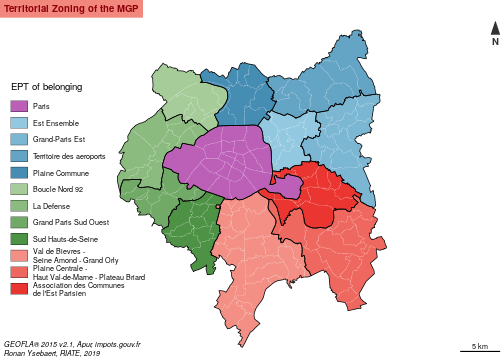The 150 municipalities of the MGP are grouped in 12 intermediate territorial divisions: the Établissements Publics Territoriaux (EPT). This territorial zoning respect approximately the delineation of the départements : the EPT of Seine-Saint-Denis are displayed in blue palette; the EPT of Paris is displayed in purple; the EPT of Hauts-de-Seine are displayed in green palette (it includes also one municipality of Val-d'Oise in the North-West of Boucle Nord 92) and the EPT of Val-de-Marne are displayed in red palette (it includes also 6 municipalities in the south part of Val-de-Bièvres EPT).

## 3.2 Numerator (amount of income tax reference) and Denominator (number of tax households)

First it is interesting to plot on maps the different statistical dimensions of the indicator we are interested in: the numerator (total amount of income tax references), the denominator (number of tax households) and the ratio (average income per tax households).

``````# layout
par(mfrow = c(1, 2), mar = c(0, 0, 1.2, 0))

# numerator map
com\$INCM <- com\$INC / 1000000
plot(st_geometry(com),  col = "peachpuff",  border = NA)
plot(st_geometry(ept),  col = NA,  border = "black", add = TRUE)

propSymbolsLayer(x = com,
var = "INCM",
symbols = "circle", col =  "#F6533A",
inches = 0.15,
border = "white",
legend.pos = "topleft", legend.values.rnd = 0,
legend.title.txt = "Amount of income taxe reference\n(millions of euros)",
legend.style = "c")
# layout
layoutLayer(title = "Numerator - Amount of income tax reference",
sources = "GEOFLA® 2015 v2.1, Apur, impots.gouv.fr",
north = FALSE, scale = FALSE, tabtitle = TRUE, frame = F, theme = "red.pal",
author = "Ronan Ysebaert, RIATE, 2019")

# denominator map
plot(st_geometry(com),  col = "peachpuff",  border = NA)
plot(st_geometry(ept),  col = NA,  border = "black", add = TRUE)
propSymbolsLayer(x = com,
var = "TH",
symbols = "circle", col =  "#515FAA",
border = "white",
inches = 0.15,
legend.pos = "topleft", legend.values.rnd = -2,
legend.title.txt = "Number of tax households",
legend.style = "c")

# layout
layoutLayer(title = "Denominator - Tax households",
sources = "",
north = TRUE, scale = 5, tabtitle = TRUE, frame = FALSE,
theme = "red.pal", author = "")
``````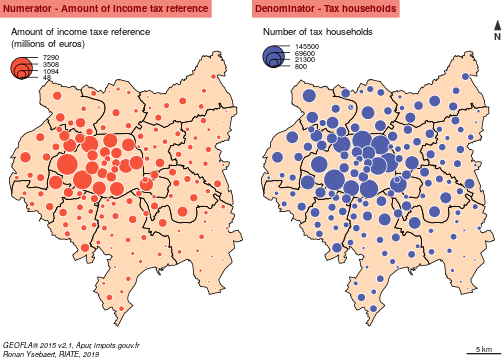Without surprise, the highest amounts of tax households and income are located in the central area of the MGP (Parisian Arrondissements). That being said, these two maps suggest an unequal distribution of income in relation to the distribution of population in Paris suburbs.

## 3.3 Ratio (average amount of income tax reference per households)

``````par(mar = c(0, 0, 1.2, 0))

# ratio
com\$ratio <- com\$INC / com\$TH

# ratio map
choroLayer(x = com,
var = "ratio",
breaks = c(min(com\$ratio, na.rm = TRUE), 20000, 30000, 40000, 50000, 60000,
max(com\$ratio, na.rm = TRUE)),
col = carto.pal(pal1 = "red.pal", n1 = 6),
border = "white",
lwd=0.2,
legend.pos = "topleft",
legend.title.txt = "Average amount of income tax\nreference per households\n(in euros)",
legend.values.rnd = 0)

# EPT borders
plot(st_geometry(ept), border = "black",add = TRUE)

# layout
layoutLayer(title = "Ratio - Income per tax households, 2013",
sources = "GEOFLA® 2015 v2.1, Apur, impots.gouv.fr",
north = TRUE, scale = 5, tabtitle = TRUE, frame = FALSE, theme = "red.pal",
author = "Ronan Ysebaert, RIATE, 2019")
``````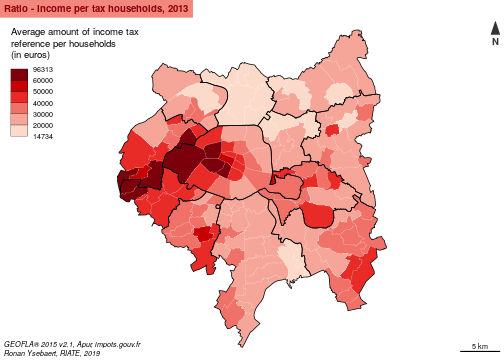The MGP area is characterized by high income inequalities. For the 150 municipalities of this area, the values extend from 14 730 (La Courneuve) to 96 310 euros (Paris, 7th Arrondissement). 53 municipalities of the MGP area (35 % of the municipalities) are below the French average, i.e. 25 660 euros. The lagging households are mainly concentrated into the north part of MGP area. Highest values are concentrated in the Western part of Paris and its suburbs.

# 4. Introducing the MTA Functions

## 4.1 General, territorial and spatial deviations

`MTA` package introduces three contexts to monitor territorial inequalities: the general deviation, the territorial deviation and the spatial deviation.

The global deviation is dedicated to the analysis of inequalities using a value of reference. In this example the global deviation refers to the inequalities existing between each municipality in regard to the whole MGP value.

The territorial deviation consists in measuring the inequalities existing for each basic territorial unit in regard to an intermediate territorial level of reference. In this case-study, for each basic territorial unit (municipalities of the MGP in this case), it implies to include beforehand in the input dataset a territorial a factor describing the intermediate territorial belonging (departements or EPT). It allows to measure for each municipality the deviation existing as regards to their EPT or departement of belonging.

The spatial deviation is a measure of inequalities taking into account the neighborhood as a reference. It allows to measure the deviation existing between basic territorial units using three possible parameters : the territorial contiguity (order 1, 2 or n), the spatial neighborhood (territorial units located at less than X kilometers as the crow flies) or functional distances (territorial units located at less than Y minutes by road for instance). In `MTA`, territorial contiguity and spatial neighborhood measures are calculated directly from an sf object. Functional distances must be uploaded separately through a dataframe structured with the following fields : id1, id2, distance measure. For this case-study, the contiguity criteria (order 1) has been retained for the calculation of the spatial deviation. It gives a good proxy of proximity relationships according to the size and the homogeneity of the municipalities of the MGP area. Other measures could be also adapted, such as time-distances by road (municipalities located at less than 15 minutes by car) or by public transport.

## 4.2 Relative and absolute deviations

In MTA, two methods are implemented to measure statistical differences to a given context of reference: the relative deviation and the absolute deviation. In `MTA`, each indicator is considered as a ratio defined by a numerator (GDP for instance) divided by a denominator (population for instance).

The relative deviation states the position of each region as regard to a context of reference expressed in index 100. It is based on the following calculation: Relative deviation (Region i) = 100 * ((Numerator(Region i)/Denominator(Region i)) /(reference ratio)) Territorial units characterized by a context of reference below index 100 are under the average of a given context of reference, and reciprocally.

The absolute deviation specifies which process of redistribution should be realized in absolute terms in order to achieve perfect equidistribution of the ratio of reference in the global, the territorial or the spatial context. It is calculated as below: Absolute deviation (Region i) = Numerator (Region i) - (reference ratio * denominator (Region i)) It examines how much amount of the numerator should be moved in order to reach equidistribution, for each territorial unit, taking into account as a reference the selected deviation context value. More generally, absolute deviation must be considered as a statistical tool to discuss on the amplitude of existing territorial inequalities in absolute terms. It is obvious that reaching a perfect equilibrium between territorial units is highly sensitive and is scarcely a policy objective itself. It is nevertheless interesting to consider the amount of money or population affected by the inequality to have in hand a concrete material for leading discussions on this delicate spatial planning issue.

## 4.3 Cartography of relative and absolute deviations

In this vignette, color palette proposed for displaying on map the relative deviations are the ones suggested by the HyperAtlas tool (blue palette = under the average; red palette = above the average). But other diverging palettes (green/red, etc.) could be also be used for displaying MTA results on maps.
Absolute deviations are highlighted using proportional circles on maps. It examines which amount of the numerator should be moved to the poorest municipalities to reach equidistribution. Circles displayed in a red palette means that the territorial unit have to contribute a given amount of numerator to achieve the equilibrium in a given context; and reciprocally circles displayed in a blue palette means that the territorial unit have to receive a given amount of numerator to achieve perfect convergence.

In this example, we have decided to merge in a same map the relative deviation (color of the circles) and the absolute deviation (size of the circles) to be more synthetic. In HyperAtlas tool, this map is split in two maps (one for the relative and one for the absolute deviation). It is quite easy to reproduce these maps using the functionalities of the `cartography` package.

## 4.4 Synthesis

The analysis of the three deviations provides generally a high number of information, difficult to synthesize. The synthesis functions helps to summarize values of the global, territorial and spatial deviations and allow to answer to some basic and interesting questions:

• Which territorial units are positioned in a favorable situation for the three deviations?
• Which territorial units are positioned in a lagging situation for the three deviations?
• Which territorial units are positioned in a contradictory situation? It means that a territorial unit is situated above a given threshold for a context and under the same threshold for the other contexts. This situation is especially interesting from a social and policy points of view (NIMBY phenomena, characterizing the opposition by residents to a proposal for a new development because it is close to them).

# 5. Global deviation

This part proposes some graphical outputs helping to have an idea regarding inequalities existing at the global level. In this case, for all the study area: the Metropole du Grand Paris.

## 5.1 Global deviation and theoretical redistribution

The code below takes in entry the numerator (INC) and the denominator (TH) of the com object and returns the global deviation indicators using `gdev()` function. The relative deviation (`type = "rel"`) and the absolute one (`type = "abs"`) are computed. These indicators are afterwards mapped.

``````# general relative deviation
com\$gdevrel <- gdev(x = com,
var1 = "INC",
var2 = "TH",
type = "rel")

# general absolute deviation
com\$gdevabs <- gdev(x = com,
var1 = "INC",
var2 = "TH",
type = "abs")

# general deviation in million Euros
com\$gdevabsmil <- com\$gdevabs / 1000000

# margins
par(mar = c(0, 0, 1.2, 0))

# Plot territories
plot(st_geometry(com), col = "grey70", border = "#EDEDED", lwd = 0.25)
plot(st_geometry(ept), border = "#1A1A19", lwd = 1, add = TRUE)

# Global deviation (relative and absolute) cartography
propSymbolsChoroLayer(x = com,
var = "gdevabsmil", var2 = "gdevrel",
inches = 0.3,
col = carto.pal(pal1 = "blue.pal", n1 = 3,
pal2 = "wine.pal", n2 = 3),
breaks = c(min(com\$gdevrel, na.rm = TRUE),
75, 90, 100, 111, 133,
max(com\$gdevrel, na.rm = TRUE)),
border = "#f0f0f0",
lwd = 0.25,
legend.var.pos = "left", legend.var2.pos = "topleft",
legend.var.title.txt = "Redistribution (Million euros)",
legend.var2.title.txt = "Deviation to the global context (100 = Metropole du Grand Paris average)",
legend.var.style = "e",
legend.var.values.rnd = 0,
legend.var2.values.rnd = 0)

# layout
layoutLayer(title = "Global deviation - Tax income per households",
sources = "GEOFLA® 2015 v2.1, Apur, impots.gouv.fr",
north = TRUE, scale = 5, tabtitle = TRUE, frame = FALSE, theme = "red.pal",
author = "Ronan Ysebaert, RIATE, 2019")
``````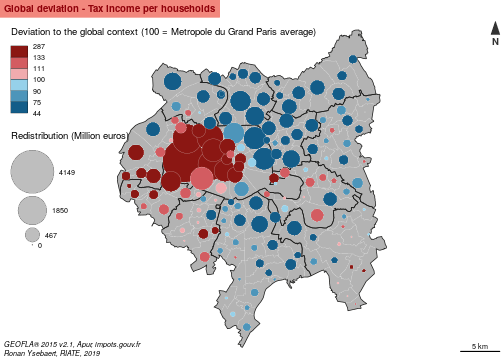The resulting map highlights strong statistical differences of income earning in the MGP area (color of the circles). It is firstly interesting to know that all the municipalities of the EPT of Plaine Commune, Territoire des Aéroports and Est Ensemble are below the average of the MGP area (33 501 euros per households). For the territories of Val de Bièvres and Grand-Paris Est, only three municipalities are above the average of the study area. Reversely, all the municipalities of the Grand-Paris-Sud-Ouest EPT are largely above the average of the MGP area. For the other EPT the situation is mixed, depending of the municipalities.

This map and these tables highlight the municipalities which may have to contribute / receive the highest to ensure a perfect equidistribution in the context of the MGP (size of the circles). In this example, the 7th Arrondissement of Paris is the municipality which should contribute the most, all things being equal to its income tax level (1,987 billion Euros of income transfer, 65 % of the total amount of tax income in this municipality). Neuilly-sur-Seine (third position in absolute terms) should transfer 1,9 billion Euros to the lagging municipalities of the MGP area. It corresponds to 62,87% of the total amount of tax income declared in this municipality.

In the other side of the redistribution, La Courneuve should receive 402 million Euros from the wealthiest municipalities (127 % of the current tax income declared). Aubervilliers should receive 793 million Euros, which represents 124 % of the current tax income in this municipality.

The code below allows to order the municipalities that have to receive / contribute the most in relative term in the global context to ensure a perfect equilibrium of resources.

``````# general deviation - Top 10 of the potential contributors in regard
# Drop geometries
df <- st_set_geometry(com, NULL)

# to their total amount of income
df\$gdevabsPerc <- df\$gdevabs / df\$INC * 100
df <- df[order(df\$gdevabsPerc, decreasing = TRUE), ]
df[1:10, c("gdevabsmil","gdevabsPerc")]
``````
``````##                          gdevabsmil gdevabsPerc
## Paris 7e Arrondissement  1987.18261    65.21643
## Marnes-la-Coquette         46.58689    63.53843
## Neuilly-sur-Seine        1900.79972    62.87958
## Paris 8e Arrondissement  1167.45176    59.37545
## Paris 16e Arrondissement 4148.92114    56.91599
## Paris 6e Arrondissement  1030.25933    55.83634
## Vaucresson                174.81548    54.71988
## Saint-Cloud               473.60724    48.07040
## Ville-d'Avray             165.60016    46.23143
## Garches                   246.70718    43.19362
``````
``````# general deviation - Top 10 of the potential receivers in regard to
# their total amount of income
df <- df[order(df\$gdevabsPerc, decreasing = FALSE), ]
df[1:10, c("gdevabsmil", "gdevabsPerc")]
``````
``````##                          gdevabsmil gdevabsPerc
## La Courneuve              -402.2023  -127.37566
## Aubervilliers             -793.0032  -124.08051
## Clichy-sous-Bois          -243.2493  -114.90485
## Bobigny                   -465.2779  -108.01736
## Stains                    -313.7436  -105.88273
## Villetaneuse              -104.6940   -99.97363
## Saint-Denis               -941.8914   -93.24860
## Pierrefitte-sur-Seine     -237.5380   -92.02924
## Villeneuve-Saint-Georges  -279.8035   -85.87404
## Dugny                      -81.3603   -83.81888
``````

## 5.2 Lorenz Curve and inequality indexes

The `ineq` package proposes some functions useful for depicting global inequalities existing in a study area. The Lorenz-curve was developed first by Max O. Lorenz in 1905 as a graphical representation of income distribution. The Lorenz Curve function takes in entry the numerator and the denominator and returns a Lorenz Curve plot; inequality indexes take in entry the ratio (numerator / denominator) and returns econometric indexes of inequality.

``````# Lorenz Curve
par(mar=c(4, 4, 4, 4))
par(pty = "s")

plot(Lc(com\$INC, com\$TH),
panel.first = grid(10, 10),
main = "Lorenz Curve - Income distribution",
xlab = "Percent of income (cumulative)",
ylab = "Percent of households",
ylim = c(0,1),
xlim = c(0,1),
asp = 1)
``````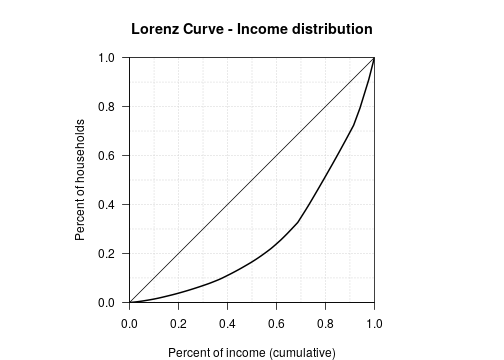``````# Inequality indexes (Gini, standard deviation and cofficient of variation)
Gini(com\$ratio)
``````
``````##  0.2299973
``````
``````sd(com\$ratio, na.rm = TRUE)
``````
``````##  15362.18
``````
``````sd(com\$ratio, na.rm = TRUE)/mean(com\$ratio, na.rm = TRUE) * 100
``````
``````##  45.67906
``````

The curve depicts on its horizontal axis a defined population – e.g., all households – broken down into deciles and ordered from left to right on the horizontal axis, from the lower tax income per household to the higher. On the vertical axis of the Lorenz curve is shown the cumulative percentage of tax income.

This plot reveals these following configurations as regard to social household distribution in MGP:

• 50 % of the households earns less than 25 % of the total income.
• 50 % of the total income is held by less than 18 % of households.

The analysis of Gini and variation coefficient give a global overview of the degree of inequality. The Gini index is comprised between 0 (equidistribution) and 1 (maximal concentration). But more interesting is analysis of the evolution of these indexes over the time (more equality? less inequality?)

# 6. Territorial deviation

This part proposes some graphical representations helping to have an idea regarding inequalities existing at territorial level. In this case, as regard to the average of each EPT (EPT).

## 6.1 Territorial deviation and theoretical redistribution

The code below takes in entry the numerator (INC) and the denominator (TH) of the com object and returns the territorial deviation indicators (relative and absolute) using the `tdev()` function. These indicators are afterwards mapped.

``````# Territorial relative deviation calculation
com\$mdevrel <- tdev(x = com,
var1 = "INC",
var2 = "TH",
type = "rel",
key = "LIBEPT")

# Territorial absolute deviation calculation
com\$mdevabs <- tdev(x = com,
var1 = "INC",
var2 = "TH",
type = "abs",
key = "LIBEPT")

# Territorial deviation in million Euros
com\$mdevabsmil <- com\$mdevabs / 1000000

# Cartography
# Plot layout
par(mfrow = c(1, 1), mar = c(0, 0, 1.2, 0))

# Plot territories
plot(st_geometry((com)), col = "grey70", border = "#EDEDED",lwd = 0.25)
plot(st_geometry((ept)), border = "#1A1A19", lwd = 1, add = T)

# Territorial deviation (relative and absolute) cartography
propSymbolsChoroLayer(x = com,
var = "mdevabsmil", var2 = "mdevrel",
inches = 0.3,
col = carto.pal(pal1 = "blue.pal", n1 = 3,
pal2 = "wine.pal", n2 = 3),
breaks = c(min(com\$mdevrel, na.rm = TRUE),
75, 90, 100, 111, 133,
max(com\$mdevrel, na.rm = TRUE)),
border = "#f0f0f0",
lwd = 0.25,
legend.var.pos = "left", legend.var2.pos = "topleft",
legend.var.title.txt = "Redistribution (Million euros)",
legend.var2.title.txt = "Deviation to the territorial context (100 = EPT average)",
legend.var.style = "e",
legend.var.values.rnd = 0,
legend.var2.values.rnd = 0)

# layout
layoutLayer(title = "Territorial deviation - Tax income per households, 2013",
sources = "GEOFLA® 2015 v2.1, Apur, impots.gouv.fr",
north = TRUE, scale = 5, tabtitle = TRUE, frame = FALSE, theme = "red.pal",
author = "Ronan Ysebaert, RIATE, 2019")
``````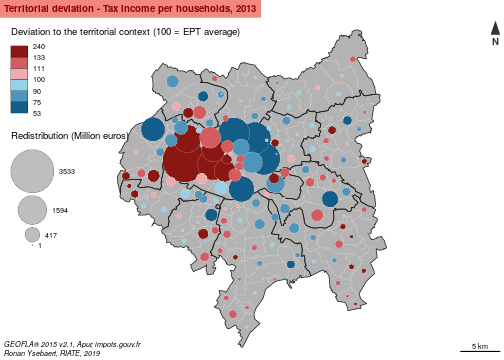The map highlight important statistical differences in each EPT in relative terms. The strongest differences in relative terms are located in Paris (opposition between the eastern part and the western part of this EPT) and in the Plaine centrale - Haut Val de Marne EPT (opposition between the poorest municipalities located near Paris and the ones located in the periphery). Globally, the richest and the poorest EPT ( Grand Paris Sud Ouest / Plaine Commune and Territoires des Aéroports du Nord Ouest) appear relatively homogeneous statistically. In other EPT, one municipality appears largely above the average of their EPT of belonging. It is the case in Est Ensemble (Les Lilas), Boucle-Nord 92 (Bois-Colombes), Sud Hauts-de-Seine (Sceaux).

The circles highlight the municipalities which may have to contribute (red palette) / receive (blue palette) the highest to ensure a perfect equilibrium of income per household for each EPT. The 7th Arrondissement of Paris is the municipality which should contribute the most to the poorest municipalities of Paris as regards to the amount of income available in this municipality (1,779 billion Euros of income transfer, 58 % of the amount of income in this municipality). Marnes-La-Coquette (second position) should transfer 38 million Euros to the poorest municipalities of its EPT of belonging (La Défense). It is relatively low as regards to the 7th Arrondissement of Paris, but it corresponds to 52 % of the total amount of income available in this municipality.

From the other side of the redistribution, Nanterre, Clichy-sous-Bois and the 19th Arrondissement of Paris should receive respectively 1088, 143 and 1926 million euros from the richest municipalities of their EPT of belonging. It represents respectively 88 %, 68 % and 65 % of the total amount of earned income of their households. The highest redistribution for this study area stands for the 20th Arrondissement of Paris (1,926 billion euros, 59 % of its total amount of income).

``````# general deviation - Top 10 of the potential contributors in regard
# Drop geometries
df <- st_set_geometry(com, NULL)

# Territorial deviation - Top 10 of the potential contributors as regards to their total amount of income
df\$mdevabsPerc <- df\$mdevabs / df\$INC * 100
df <- df[order(df\$mdevabsPerc, decreasing = TRUE), ]
df[1:10, c("mdevabsmil", "mdevabsPerc")]
``````
``````##                          mdevabsmil mdevabsPerc
## Paris 7e Arrondissement  1779.21614    58.39127
## Marnes-la-Coquette         37.85668    51.63157
## Paris 8e Arrondissement  1010.71931    51.40419
## Neuilly-sur-Seine        1468.33529    48.57340
## Paris 16e Arrondissement 3532.67330    48.46214
## Paris 6e Arrondissement   870.36502    47.17065
## Santeny                    37.74031    43.38812
## Marolles-en-Brie           47.14636    42.74486
## Vaucresson                119.06443    37.26896
## Le Raincy                 116.35420    35.78738
``````
``````# Territorial deviation - Top 10 of the potential receivers as regards to their total amount of income
df <- df[order(df\$mdevabsPerc, decreasing = FALSE), ]
df[1:10, c("mdevabsmil", "mdevabsPerc")]
``````
``````##                          mdevabsmil mdevabsPerc
## Nanterre                 -1087.5931   -88.08889
## Clichy-sous-Bois          -143.1380   -67.61479
## Paris 19e Arrondissement -1925.8379   -65.44786
## Paris 20e Arrondissement -1958.2712   -59.73389
## Bagneux                   -295.2509   -58.59873
## Paris 18e Arrondissement -1864.1104   -53.46040
## Champigny-sur-Marne       -509.8546   -49.20335
## Gennevilliers             -197.9141   -43.87097
## Villeneuve-Saint-Georges  -123.2206   -37.81744
## Paris 13e Arrondissement -1241.4815   -36.30654
``````

## 6.2 Box-plot by Établissement Public Territorial

Another way to explore characteristics of the territorial deviation consists in analyzing the statistical dispersion (general deviation) by intermediate level (EPT in this case). The best suited graphical representation for this kind of analysis is certainly the boxplot.

The code below takes in entry the general deviation calculated above and the intermediate levels included in the input dataset (com). It returns a boxplot displaying the statistical parameters (median, mean, 1st and 3rd quartiles, range, minimum and maximum, extraordinary values) allowing to observe the statistical dispersion existing for each intermediate zoning (in this case each EPT). To ease the interpretation and the synthesis of the plot, boxplots are ordered by mean values for each intermediate levels. Moreover, the width of the bars are proportional to the number of territorial units included in each intermediate zoning.

``````par(cex.lab = 1)
par(cex.axis = 0.75)
par(mar = c(4, 4, 2, 2))

# Drop geometries
df <- st_set_geometry(com, NULL)

# Reorder EPT according to gdev value
df\$EPT <- with(df, reorder(EPT, gdevrel, mean, na.rm = TRUE))

# Colors management
col <- carto.pal(pal1 = "red.pal", n1 = (nlevels(df\$EPT) / 2),
pal2 = "green.pal", n2 = (nlevels(df\$EPT) / 2),
middle = FALSE, transparency = TRUE)

# Boxplot
boxplot(df\$gdevrel ~ df\$EPT,
col = col,
ylab = "Global deviation",
xlab = "Territorial deviation",
varwidth = TRUE,
range = 1,
outline = TRUE,
las = 1)

# Horizontal Ablines
abline (h = seq(40, 300, 10), col = "#00000060", lwd = 0.5, lty = 3)

# Plot mean values
xi<- tapply(df\$gdevrel, df\$EPT, mean, na.rm = TRUE)
points(xi, col = "#7C0000", pch = 19)

# Legend for the boxplot
df\$EPTName<- as.factor(df\$LIBEPT)
df\$EPTName <- with(df, reorder(EPTName, gdevrel, mean, na.rm = TRUE))
legend("topleft",
legend = levels(df\$EPTName),
pch = 15,
col = col,
cex = 0.6,
pt.cex = 1,
title = "Territorial contexts (ordered by mean value of global deviation)")
``````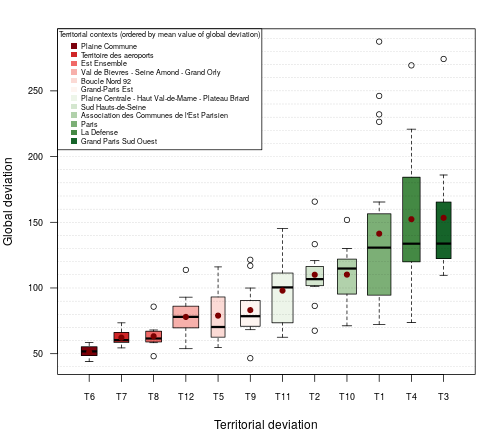This plot highlights the statistical dispersion existing in each EPT. It completes the analysis proposed in the previous map. This boxplot delivers several learning: Firstly, it confirms globally that wealthier the EPT is, larger the statistical differences between the poorest and the wealthiest territorial units are. In this perspective, Plaine Commune and Territoire des Aéroports (T6 and T7) are quite homogeneous (all the municipalities of these territories are lagging). On the reverse, the Arrondissements of Paris are characterized by strong differences between minimum (index 71) and maximum values (index 290). The same is true with La Defense and Grand Paris Sud Ouest (T4 and T3) with important interquartile values. Secondly, extraordinary values (dots out of the box) concerns poor and wealthy EPT. But for this study area outliers mainly concern maximum values, especially for Paris, La Défense, Grand Paris Sud Ouest and ACEP. Finally, it is interesting to note that Est Ensemble, Grand Paris Est and Sud Hauts-de-Seine (EPT T8, T9 and T2) include municipalities with very low average income per household, since they are characterized by outliers in the low values.

# 7. Spatial deviation

This part proposes some graphical representations helping to have an idea regarding inequalities existing in a local (or spatial) context. In this case, as regard to the average of contiguous territorial units (contiguity order 1).

## 7.1 Spatial deviation and theoretical redistribution

The code below takes in entry the numerator (INC) and the denominator (TH) of the com object and returns the spatial deviation indicators (relative and absolute) using the `sdev()` function. These indicators are afterwards mapped.

``````# Spatial relative deviation calculation
com\$ldevrel <- sdev(x = com, xid = "DEPCOM", var1 = "INC", var2 = "TH",
order = 1, type = "rel")

# Spatial absolute deviation calculation
com\$ldevabs <- sdev(x = com, xid = "DEPCOM", var1 = "INC", var2 = "TH",
order = 1, type = "abs")

# Spatial deviation in million Euros
com\$ldevabsmil <- com\$ldevabs / 1000000

# Cartography
# Plot layout
par(mfrow = c(1, 1), mar = c(0, 0, 1.2, 0))

# Plot territories
plot(st_geometry(com), col = "grey70", border = "#EDEDED",lwd = 0.25)
plot(st_geometry(ept), border = "#1A1A19",lwd = 1, add = T)

# Territorial deviation (relative and absolute) cartography
propSymbolsChoroLayer(x = com,
var = "ldevabsmil", var2 = "ldevrel",
inches = 0.3,
col = carto.pal(pal1 = "blue.pal", n1 = 3,
pal2 = "wine.pal", n2 = 3),
breaks = c(min(com\$ldevrel, na.rm = TRUE),
75, 90, 100, 111, 133,
max(com\$ldevrel, na.rm = TRUE)),
border = "#f0f0f0",
lwd = 0.25,
legend.var.pos = "left", legend.var2.pos = "topleft",
legend.var.title.txt = "Redistribution (Million euros)",
legend.var2.title.txt = "Deviation to the spatial context
(100 = average of the contiguous territorial units - order 1)",
legend.var.style = "e",
legend.var.values.rnd = 0,
legend.var2.values.rnd = 0)

# layout
layoutLayer(title = "Spatial deviation - Tax income per households, 2013",
sources = "GEOFLA® 2015 v2.1, Apur, impots.gouv.fr",
north = TRUE, scale = 5, tabtitle = TRUE, frame = FALSE, theme = "red.pal",
author = "Ronan Ysebaert, RIATE, 2019")
``````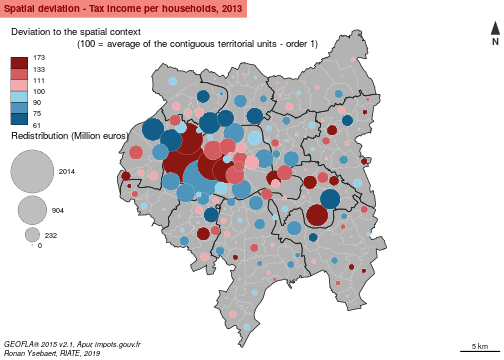This map highlights local discontinuities existing in the MGP. Important local statistical gaps appear in several areas: Saint-Mandé and Neuilly-sur-Seine are characterized by the highest score as regards to their respective neighbors (indexes 173 and 156). The central Arrondissements of Paris (6e, 7e, 8e) appear also in favorable situation in a local context. Some local “bastions” are revealed in the periphery of Paris, such as Le Raincy (index 153), Sceaux (150), Vaucresson (143), Marnes-la-Coquette (142) or Saint-Maur-des-Fossés (140). Lagging municipalities are mainly located in the near periphery of Paris: the lower index (61) is observed at Clichy-sous-Bois. Clichy, Puteaux, Saint-Ouen, Bagneux and Aubervilliers are also in a lagging situation: their average income per household stand around 30-40 % below their respective neighborhood.

Looking into local redistributions, the most part of the redistribution in absolute terms concerns the Arrondissements of Paris and their contiguous municipalities in the north-west of Paris: the 16th Arrondissement of Paris should contribute to 2 billion euros; the 15th Arrondissement should receive 1,5 billion euros to ensure a local equilibrium.
But as regard to the available income mass (table below), it is Saint-Mandé (260 million euros, 42 % of the total income of the municipality), Neuilly-sur-Seine (1 billion euros, 36 %) and the 6th Arrondissement (650 million, 35 %) which should contribute to their respective poorest neighbors. On the other side of the redistribution, Clichy-sous-Bois (138 million euros, 65 %), Clichy (521 million, 62 %), Puteaux (535 million, 58 %) and Saint-Ouen (311 million, 56 %) should receive the most. Interesting is to note that the local redistribution implies a lowest share of their respective income for the wealthiest municipalities (around 35 %) comparing to the poorest one (which should receive more than 50 % of their current available income).

``````# Drop geometries
df <- st_set_geometry(com, NULL)

# Spatial deviation - Top 10 of the potential contributors as regards to their total amount of income
df\$ldevabsPerc<- df\$ldevabs / df\$INC * 100
df<-df[order(df\$ldevabsPerc, decreasing = TRUE), ]
df[1:10, c("ldevabsmil","ldevabsPerc")]
``````
``````##                         ldevabsmil ldevabsPerc
## Saint-Mande              260.10243    42.35190
## Neuilly-sur-Seine       1090.39208    36.07081
## Paris 6e Arrondissement  650.36361    35.24736
## Le Raincy                112.64658    34.64702
## Paris 7e Arrondissement 1052.81249    34.55177
## Sceaux                   187.23855    33.59400
## Gournay-sur-Marne         45.78709    32.77097
## Coubron                   25.56596    31.16313
## Vaucresson                95.59513    29.92272
## Marnes-la-Coquette        21.91940    29.89520
``````
``````# Spatial deviation - Top 10 of the potential receivers as regards to their total amount of income
df<-df[order(df\$ldevabsPerc, decreasing = FALSE), ]
df[1:10, c("ldevabsmil","ldevabsPerc")]
``````
``````##                     ldevabsmil ldevabsPerc
## Clichy-sous-Bois    -138.01472   -65.19469
## Clichy              -521.27310   -62.11944
## Puteaux             -535.70339   -58.26476
## Saint-Ouen          -311.73019   -56.39945
## Bagneux             -249.46913   -49.51238
## Aubervilliers       -303.14284   -47.43249
## Bonneuil-sur-Marne   -84.77851   -46.04200
## Nanterre            -556.15780   -45.04563
## Champigny-sur-Marne -456.19566   -44.02501
## Gennevilliers       -182.72267   -40.50353
``````

## 7.2 Spatial autocorrelation plot and outliers analysis

Spatial autocorrelation reveals the absence of independence between geographical observations. Thus, spatialized indicators are often subject to spatial dependencies (or spatial interactions), which are even stronger than spatial localizations are closer. Autocorrelation measures (Moran, Geary, Lisa indexes) allows estimating the spatial dependence between the values of a same indicator at several locations of a given study area.

The figure displayed below consists in evaluating this spatial autocorrelation by a plot crossing the spatial deviation (Y axis) and global deviation (X axis) values. It includes also a regression line, the equation and the R-Squared of this statistical model. On top of that, colors are affected to depict the territorial level belonging, following the logic of the MTA framework.

For each territorial unit of the study area, this plot crosses the values of the global deviation on horizontal axis with the values of the local deviation on Y axis. This chart is interesting as it reveals spatial dependency, e.g. spatial organization of a phenomena. It provides some interesting inputs for answering to basic questions, such as:

• Are territorial units globally advantaged also advantaged at local level (and reciprocally)?
• Is it possible to detect special situations as regard to the global trend, meaning the existence of “local bastions” in favorable or lagging situations?
``````par(cex.lab = 1)
par(cex.axis = 0.75)
par(mar = c(4, 4, 2, 2))

# Drop geometries
df <- st_set_geometry(com, NULL)

# label order
df\$LIBEPT <- as.factor(df\$LIBEPT)
df\$LIBEPT <- ordered(df\$LIBEPT,
levels = c("Paris",
"Est Ensemble",
"Grand-Paris Est",
"Territoire des Aéroports",
"Plaine Commune",
"Boucle Nord 92",
"La Defense",
"Grand Paris Sud Ouest",
"Sud Hauts-de-Seine",
"Val de Bievres - Seine Amond - Grand Orly",
"Plaine Centrale - Haut Val-de-Marne - Plateau Briard",
"Association des municipalities de l'Est Parisien"))

# colors
df\$col <- as.factor(df\$LIBEPT)
levels(df\$col) <- cols

# Spatial autocorrelation
lm <- summary.lm(lm(ldevrel ~ gdevrel, df))

# Plot spatial autocorrelation
plot(df\$gdevrel, df\$ldevrel,
ylab = "Local deviation",
ylim = c(50,260),
xlab = "Global deviation",
xlim = c(50,260),
pch = 20,
col = as.vector(df\$col),
asp = 1)
abline((lm(df\$ldevrel ~ df\$gdevrel)), col = "red", lwd =1)

# Specify linear regression formula and R-Squared of the spatial autocorrelation on the plot
text(140,65, pos = 4, cex = 0.8,
labels = (paste("Local Deviation =", round(lm\$coefficients["gdevrel","Estimate"], digits = 3),
"* (Global Deviation) +", round(lm\$coefficients["(Intercept)","Estimate"],
digits = 3))))
text(140,55, pos = 4, cex = 0.8,
labels = (paste("R-Squared =", round(summary(lm(ldevrel~gdevrel, com ))\$r.squared, digits = 2))))

abline (h = seq(40,290,10), col = "gray70", lwd = 0.25, lty = 3)
abline (h = seq(50,250,50), col = "gray0", lwd = 1, lty = 1)
abline (v = seq(40,290,10), col = "gray70", lwd = 0.25, lty = 3)
abline (v = seq(50,250,50), col = "gray0", lwd = 1, lty = 1)

# Legend for territorial level
legend("topleft",
legend = levels(df\$LIBEPT),
pch = 20,
col = cols,
cex = 0.6,
pt.cex = 1,
title = "Territorial context")
``````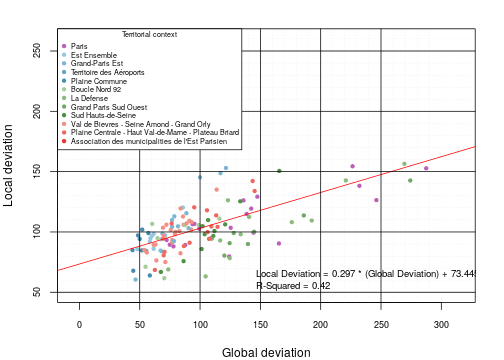The output of the linear model of spatial autocorrelation reveals that the hypothesis of independence is rejected at a probability below than 0.0001. It means that, “everything is related to everything else, but near things are related than distant things” (Tobler, 1970). However, the R-squared of the relation (0.42) suggests that this statistical relation includes outliers very far from the linear regression, which may be interesting to analyze. On the plot, it is possible to visualize then municipalities, which mainly belong to Seine-Saint-Denis (Grand-Paris Est).

More empirically, the chart can also be used to examine the situation of outliers and exceptional units out of the cloud of points. The code below takes in entry the statistical residuals of the spatial autocorrelation calculated in the previous section. Then the residuals are standardized. The code returns a plot displaying the standardized residuals according to the value of the local deviation. Exceptional values (calculated using the T-Student value) are labeled on the resulting plot.

``````par(cex.lab = 1)
par(cex.axis = 0.75)
par(mar = c(4, 4, 2, 2))

# Standardized residual calculation
lm <- lm(ldevrel ~ gdevrel, df)
res.standard <- rstandard(lm)

#risk alpha = 0.1
alpha <- 0.1

# Calculation of the threshold using T-Student at (n-p-1) degrees of freedom
seuil.standard <- qt(1 - alpha / 2, nrow(com) - 1)

# Plot residuals
plot(df\$ldevrel, res.standard,
xlab = "Local deviation", cex.lab = 0.8,
xlim = c(60, 180),
ylab = "Standardized residuals of spatial autocorrelation", cex.lab = 0.8,
pch = 20,
col = as.vector(df\$col),
cex.axis = 0.8)

abline(h = -seuil.standard, col = "red")
abline(h = +seuil.standard, col = "red")
abline(h = 0, col = "red")

# Detecting exceptional values and labeling them on the plot
ab.standard <- df[res.standard < -seuil.standard | res.standard > +seuil.standard,]

for (i in 1 : nrow(ab.standard)){
# Take the territorial units listing below and above the threshold
municipalities <- row.names(ab.standard)[i]
# Plot the residual names
text(com[municipalities,"ldevrel"], res.standard[municipalities], municipalities, cex = 0.5, pos = 4)
}

abline (v = seq(50, 200, 10), col = "gray70", lwd = 0.25, lty = 3)
abline (v = seq(50, 200, 50), col = "gray0", lwd = 1, lty = 1)

# Plot the legend (territorial zoning)
legend("topleft",
legend = levels(ab.standard\$LIBEPT),
pch = 20,
col = cols,
cex = 0.6,
pt.cex = 1,
title = "Territorial context")
``````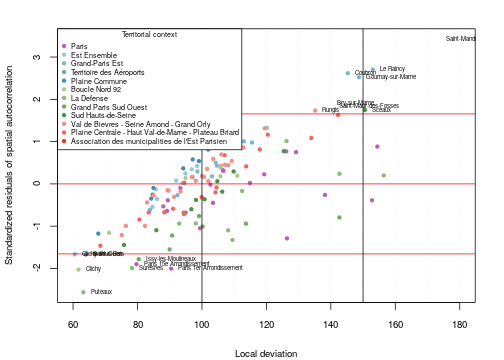This plot highlight exceptional situations, all things being equal to their situation in a global context. It depicts firstly the municipalities which are characterized by a significant lagging situation locally as regards to their average income per household (Puteaux, Clichy, Clichy-sous-Bois, Saint-Ouen, Issy-les-Moulineaux, Suresnes, Paris 1st and 15th Arrondissements). Secondly, this plot reveals also municipalities in a significantly wealthier than their neighborhoods (Saint-Mandé, Le Raincy, Coubron, Gournay-sur-Marne, Bry-sur-Marne, Saint-Maur-des-Fossés, Rungis, Sceaux). This plot is especially useful to highlight poles of wealth and poles of poverty in a local context.

## 7.3 Which value for the dist and order parameters ?

In the example above, a contiguity criteria (`order = 1`) has been chosen to evaluate inequalities at local level. This choice has been decided arbitrarily for a convenient purpose (it is easier to conceptualize the average deviation of territorial units touching themselves). But results might be significantly different using other criteria for measuring the spatial deviation.

In fact, the values of this deviation depend on some criteria:

• the intrinsic inequalities existing in the study area (the same than for the other deviations).
• the average size of territorial units (for spatial criteria): a territorial unit with a surface below 1 km square will certainly have neighbors at a threshold of 1000 m. It is no more the case for a territorial unit with a surface of 20 km square.
• the spatial extent of the study area: the spatial criteria will not be the same for a local case study, such as the municipalities of the MGP or for wider case-study (Europe for instance).

Thus, it is important for have a global overview of the effects of the neighborhood parameters (`order` and `dist` parameters) on the calculation of the spatial deviation. It is the aim of the plot below. It takes in entry a dataframe computing desired distance or contiguity levels. Using the `sdev()` function, it returns a plot showing the amount of redistribution as regard to the distance or the contiguity criteria.

``````par(cex.lab = 1)
par(cex.axis = 0.75)
par(mar = c(4, 4, 2, 2))

# Set the targeted distances for measuring spatial deviation
dfSpa <- data.frame(dist = seq(0, 35000, by = 500), ineq = NA)

# Calculation of spatial deviations in absolute terms for all the distances retained
spat <- function(x){
ldevabs <- sdev(x = com,  xid = "DEPCOM",  var1 = "INC", var2 = "TH",
dist = x, type = "abs")
ldevabspos <- ldevabs[ldevabs>0]
sum(ldevabspos)
}

dfSpa\$ineq <- apply(dfSpa, 1, spat)

# Convert results in million Euros and km
dfSpa\$ineq <- dfSpa\$ineq / 1000000
dfSpa\$dist <- dfSpa\$dist / 1000
dfSpa[is.na(dfSpa)] <- 0

# Set the targeted contiguity order for measuring spatial deviation
dfOrder <- data.frame(order = seq(0, 20, by = 1), ineq = NA)

# Calculation of spatial deviations in absolute terms for all the contiguities retained
spat <- function(x){
ldevabs <- sdev(x = com, xid = "DEPCOM", var1 = "INC", var2 = "TH", order = x,
type = "abs")

ldevabspos <- ldevabs[ldevabs>0]
sum(ldevabspos)
}

dfOrder\$ineq <- apply(dfOrder, 1, spat)

# Convert results in million Euros
dfOrder\$ineq <- dfOrder\$ineq / 1000000
dfOrder[is.na(dfOrder)] <- 0

# Combined plot - Plot 1: Euclidian Distance
plot.new()
plot.window(xlim = c(0,22500), ylim = c(0,35), xaxs = "i", yaxs = "i")
axis(1, pos = 0, xlim = c(0,22500))
axis(2, col = '#488b37', pos = 0, ylim = c(0,35))
abline (v = seq(0, 22000, 1000), col = "gray30", lwd = 0.25)
abline (h = seq(0, 35, 5), col = "#488b37", lwd = 0.25)
lines(dfSpa\$dist ~ dfSpa\$ineq, type = 'b', col = '#488b37', lwd = 0.25, lty = 1, pch = 20)
title(xlab = "Mass of numerator to redistribute required to reach the equilibrium (million Euros)")
mtext("neighborhood: Euclidian distance (km)", col = "#488b37", side = 2, line = 2)

# Adding plot 2 : contiguity
plot.window(xlim = c(0, 22500), ylim = c(0,20), xaxs = "i", yaxs = "i")
axis(4, col ='#7a378b')
abline (h = seq(0, 20, 5), col = "#7a378b", lwd = 0.25, lty = 1)
lines(dfOrder\$order ~ dfOrder\$ineq, type = 'b', col = '#7a378b', lwd = 0.25, lty = 1, pch = 20)
mtext("neighborhood: Contiguity order", side = 4, col = "#7a378b")

#title for the graph
title(main = "Numerator redistribution as regards to spatial and contiguity parameters")
box(which = "plot")
``````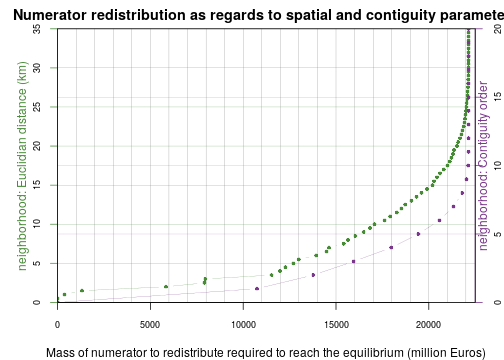If the contiguity order or spatial distance corresponds to 0, nothing happen: each territorial unit is compared to itself and it implies consequently no spatial deviation. Reversely, if these parameters are too important (above 31 km or 12 contiguity order in the case of the MGP), the spatial deviation takes for the calculation all the territorial units of the study area. In this case the values of the spatial deviation corresponds exactly to the global deviation.

In the plot displayed above, we can see that a contiguity of 1 order (chosen for this vignette) corresponds more or less 3,5 kilometers in Euclidian distance (approximately 11 billion Euros of income redistribution).

Out of these mathematical considerations related to the characteristics of the study area and the size of the elementary territorial units, it is important to remind that the choice of the appropriate threshold must make sense from a thematic point of view (does a neighborhood of 5 km mean something? Is a distance of 20 minutes by car more appropriated?)

# 8. Synthesis

All the sections above provide a high amount of information regarding territorial inequalities and potential redistributions at global, territorial and spatial contexts. This part proposes some useful plots to synthesize all this information, using the synthesis functions of the `MTA` package.

## 8.1 Synthesis of relative deviations

The three relative positions about contexts can be summarized in a synthetic typology. The elementary units can be classified in eight classes according to their three relative positions and one threshold.

Thus, the `mst()` function takes in entry all the elements required for calculating the three deviations: A sf object including the numerator and the denominator for all the deviations calculation, the “key” argument for the territorial deviation calculation and the dist/order/mat arguments for the spatial deviation calculation.

This function proposes afterwards to specify which point of view the synthesis analysis will focus on: the first “Criterion” argument (superior) shows whether the point of view is to underline the territorial units whose ratio is greater than (superior = TRUE), or to underline the territorial units below (superior = FALSE) a given threshold (threshold argument. This choice depends on the studied indicator and the aim of the analysis.

Following the HyperAtlas framework inputs, the `mst()` function returns useful elements to synthesize the value of the three deviations:

• 4 fundamental vectors are returned: the ratio (ratio), the global (gdevrel), territorial (tdevrel) and spatial (sdevrel) relative deviations.

• The synthesis of these three deviation, calculated by defining the position of the deviation according to the threshold and superior arguments. It provides a typology in 8 classes (mst column) delivering a synthesis of all possible cases: a territorial unit above/under the threshold for the 3 deviations. This is especially useful for highlighting special cases:

• A territorial unit being above/under the threshold for all the deviations (class 7). It is adapted to analyze territorial units in favorable or lagging situation.

• A territorial unit being in a contradictory situation. Two configurations may appear: a territorial unit being in a lagging situation locally (intermediate and/or spatial deviations) and in a favorable situation globally (class ); and reciprocally (classes 1,2,3,4,5,6 depending of the indicator and the threshold chosen).

• A territorial unit being out of the threshold chosen for the three deviations (class 0). In such case, it is interesting to change the threshold and the superior argument to go further in the analysis.

In the code below, we focus the analysis on municipalities above 125 % for the three deviations (gdev = Métropole du Grand Paris ; tdev = EPT average and sdev = contiguous municipalities). The `mst()` function computes the deviations and evaluate their position according to the three deviations. The subset (mst == 7) returns all the municipalities in this situation.

``````# Compute the synthesis DataFrame (relative deviations)
synthesis <- mst(x = com, var1 = "INC", var2 = "TH", key = "EPT", order = 1,
threshold = 125, superior = TRUE)
subset(synthesis, mst == 7, select = c(ratio, gdevrel, tdevrel, sdevrel, mst))
``````
``````##                             ratio  gdevrel  tdevrel  sdevrel mst
## Paris 6e Arrondissement  75856.82 226.4305 189.2887 154.4339   7
## Paris 7e Arrondissement  96313.13 287.4920 240.3342 152.7925   7
## Paris 8e Arrondissement  82465.28 246.1566 205.7791 126.4014   7
## Paris 16e Arrondissement 77757.72 232.1047 194.0321 138.1793   7
## Marnes-la-Coquette       91880.71 274.2614 206.7464 142.6436   7
## Neuilly-sur-Seine        90249.91 269.3935 194.4519 156.4231   7
## Sceaux                   55513.65 165.7067 154.8138 150.5888   7
## Vaucresson               73986.43 220.8475 159.4107 142.6996   7
## Marolles-en-Brie         48101.68 143.5822 174.6568 142.2428   7
## Saint-Mande              50852.51 151.7934 142.8341 173.4663   7
## Santeny                  48648.24 145.2137 176.6414 133.9427   7
``````
• A Suggestion of color (colors) to display on map each category of the classification. The colors proposed are the same than in the HyperAtlas tool.

### 8.1.1 municipalities in favorable situation

First of all, we aim at highlighting municipalities of the MGP in favorable situation. The `mst()` function below takes '125' for the threshold argument and 'TRUE' for the superior argument. Consequently, the typology will focus on the municipalities above 25% of the average for the three deviations. The typology is afterwards plotted using the `cartography` package.

``````par(mfrow = c(1, 1), mar = c(0, 0, 1.2, 0))

mapsynthesis <-mapmst(x = com, var1 = "INC", var2 = "TH", key = "EPT",
order = 1,threshold = 125, superior = TRUE)

layoutLayer(title = "Multiscalar synthesis - Income per household 2013",
sources = "GEOFLA® 2015 v2.1, Apur, impots.gouv.fr",
north = TRUE, scale = 5, tabtitle = TRUE, frame = FALSE, theme = "red.pal",
author = "Ronan Ysebaert, RIATE, 2019
100: Deviation average
G: Situation as compared to the global context (Grand Paris Area)
T: Situation as compared to the territorial context (EPT of belonging)
S: Sitation as compared to the neigbourhood context (contiguity order 1)")

# add labels for territorial objects above 125 % for all the deviations
labelLayer(x = mapsynthesis[ which(mapsynthesis\$mst == 7),], txt = "LIBCOM",
cex = 0.5, halo = TRUE, overlap = FALSE)
``````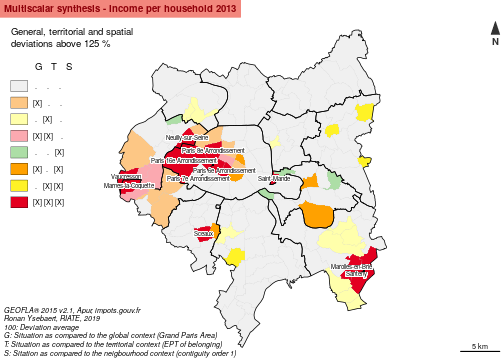This map highlights the municipalities largely above the average of the three deviations (colored in red). They are mainly located in the West part of Paris and of the MGP. Some isolated municipalities appear also locally in a favorable situation in the East Part of the MGP.

More precisely (extract of the tables below), 11 municipalities are characterized by an index of average income per household above 125% on the three contexts (class 7, red color). It corresponds to 4 Arrondissements in Paris (6e, 7e, 8e and 16e) and the municipalities of Marnes-la-Coquette, Neuilly-sur-Seine, Sceaux, Vaucresson, Marolles-en-Brie, Saint-Mandé and Santeny.

13 municipalities can be considered as “globally” advantaged (above 125% only in a global and/or in a global and in territorial contexts, colored in light orange and in pink). It corresponds to the central Arrondissements in Paris (1e, 2e, 4e, 9e) and other municipalities in favorable situation close to wealthier municipalities (Boulogne-Billancourt, Saint-Cloud, etc).

8 municipalities can be considered as “locally” advantaged (index above 125% only in a spatial context and/or in a spatial and a territorial context, colored in green and yellow). These local poles of wealth are mainly located in the periphery of the MGP area (Le Raincy, Rungis, Coubron, etc.), closed to poorer municipalities.

``````# municipalities in favorable situation for the three contexts
subset(synthesis, mst == 7, select = c(gdevrel, tdevrel, sdevrel, mst))
``````
``````##                           gdevrel  tdevrel  sdevrel mst
## Paris 6e Arrondissement  226.4305 189.2887 154.4339   7
## Paris 7e Arrondissement  287.4920 240.3342 152.7925   7
## Paris 8e Arrondissement  246.1566 205.7791 126.4014   7
## Paris 16e Arrondissement 232.1047 194.0321 138.1793   7
## Marnes-la-Coquette       274.2614 206.7464 142.6436   7
## Neuilly-sur-Seine        269.3935 194.4519 156.4231   7
## Sceaux                   165.7067 154.8138 150.5888   7
## Vaucresson               220.8475 159.4107 142.6996   7
## Marolles-en-Brie         143.5822 174.6568 142.2428   7
## Saint-Mande              151.7934 142.8341 173.4663   7
## Santeny                  145.2137 176.6414 133.9427   7
``````
``````# municipalities in favorable situation in a global context or in a global and in an territorial contexts
subset(synthesis, mst == 1 | mst == 3, select = c(gdevrel, tdevrel, sdevrel, mst))
``````
``````##                           gdevrel   tdevrel   sdevrel mst
## Paris 1er Arrondissement 165.4262 138.29105  90.43753   3
## Paris 2e Arrondissement  125.4070 104.83630 103.42912   1
## Paris 4e Arrondissement  138.5607 115.83230 114.88557   1
## Paris 9e Arrondissement  142.4046 119.04570 119.33353   1
## Paris 17e Arrondissement 144.2828 120.61579  99.37917   1
## Boulogne-Billancourt     139.6822 105.29659  89.95896   1
## Garches                  176.0366 127.06560 108.12517   3
## Levallois-Perret         133.7036  96.50905  98.09806   1
## Meudon                   127.8880  96.40575  99.10597   1
## Rueil-Malmaison          140.6932 101.55429 112.46847   1
## Saint-Cloud              192.5684 138.99850 109.47437   3
## Sevres                   144.8624 109.20161 100.19356   1
## Ville-d'Avray            185.9822 140.19896 113.65608   3
``````
``````# municipalities in favorable situation in a spatial context or in a spatial and a territorial context
subset(synthesis,  mst == 4 | mst == 6, select = c(gdevrel, tdevrel, sdevrel, mst))
``````
``````##                       gdevrel   tdevrel  sdevrel mst
## La Garenne-Colombes 123.07336  88.83603 126.2585   4
## Coubron              99.95287 128.15310 145.2710   6
## Gournay-sur-Marne   116.92059 149.90802 148.7453   6
## Le Raincy           121.46348 155.73263 153.0152   6
## Bry-sur-Marne       120.05890 112.97272 139.8264   4
## Charenton-le-Pont   114.80652 108.03034 128.4887   4
## Rungis              113.74930 153.41339 135.1303   6
## Vincennes           121.96874 114.76984 127.5193   4
``````

### 8.1.2 municipalities in lagging situation

It is also possible to analyze the reverse situation: the municipalities in lagging situation for this indicator. Thus, the `mapmst()` function takes '80' for the threshold argument and 'FALSE' for the superior argument. This typology will specify the municipalities situated below 20% of the average for the global and/or the territorial and/or the spatial contexts.

``````par(mfrow = c(1, 1), mar = c(0, 0, 1.2, 0))

mapsynthesis <- mapmst(x = com, var1 = "INC", var2 = "TH", key = "EPT",
order = 1,threshold = 80, superior = FALSE)

layoutLayer(title = "Multiscalar synthesis - Income per household 2013",
sources = "GEOFLA® 2015 v2.1, Apur, impots.gouv.fr",
north = TRUE, scale = 5, tabtitle = TRUE, frame = FALSE, theme = "red.pal",
author = "Ronan Ysebaert, RIATE, 2019
100: Deviation average
G: Situation as compared to the global context (Grand Paris Area)
T: Situation as compared to the territorial context (EPT of belonging)
S: Sitation as compared to the neigbourhood context (contiguity order 1)")

# add labels for territorial objects above 125 % for all the deviations
labelLayer(x = mapsynthesis[ which(mapsynthesis\$mst == 7),], txt = "LIBCOM",
cex = 0.6, halo = TRUE, overlap = FALSE)
``````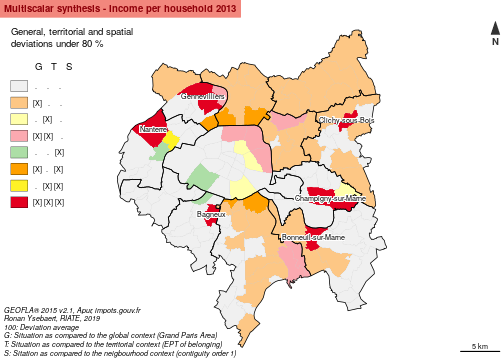The map shows the municipalities in difficulty regarding the income distribution in the MGP. Interesting is to note that all the territories of the Plaine Commune, Territoire des Aéroports EPT are below the threshold of 80 % for at least the global context. Most of the municipalities of EPT of Est Ensemble, Grand-Paris-Est, Val de Bièvres are in the same situation.

The municipalities in lagging situation for the three contexts (class 7, in red) are located in several areas of the MGP (North-West, East). All are located not in the immediate periphery of Paris (none of these municipalities are contiguous to Paris). It concerns precisely 6 municipalities: Bagneux, Genevilliers, Nanterre, Clichy-sous-Bois, Bonneuil-sur-Marne and Champigny-sur-Marne. Clichy-sous-Bois appears especially in a dramatic situation (indexes 47, 60 and 61 for the global, territorial and spatial deviations).

Most of the municipalities of Seine-Saint-Denis and Val-de-Marne are characterized by a significant lagging situation globally but not locally (classes 1 and 3, in light orange and pink). It is mainly due to the fact that the value of the index is too low to introduce significant gaps locally.

4 municipalities are characterized by low indexes in a local context, but not in a global one. It corresponds to the municipalities located in the immediate neighborhood of the wealthiest municipalities of the MGP (classes 4 and 6, in green and yellow): Paris 15e Arrondissement, Suresnes, Puteaux and in a less extent Malakoff.

``````synthesis <- mst(x = com, var1 = "INC", var2 = "TH", key = "EPT", order = 1, threshold = 125, superior = TRUE)
# municipalities in favorable situation for the three contexts
subset(synthesis, mst == 7, select = c(gdevrel, tdevrel, sdevrel, mst))
``````
``````##                           gdevrel  tdevrel  sdevrel mst
## Paris 6e Arrondissement  226.4305 189.2887 154.4339   7
## Paris 7e Arrondissement  287.4920 240.3342 152.7925   7
## Paris 8e Arrondissement  246.1566 205.7791 126.4014   7
## Paris 16e Arrondissement 232.1047 194.0321 138.1793   7
## Marnes-la-Coquette       274.2614 206.7464 142.6436   7
## Neuilly-sur-Seine        269.3935 194.4519 156.4231   7
## Sceaux                   165.7067 154.8138 150.5888   7
## Vaucresson               220.8475 159.4107 142.6996   7
## Marolles-en-Brie         143.5822 174.6568 142.2428   7
## Saint-Mande              151.7934 142.8341 173.4663   7
## Santeny                  145.2137 176.6414 133.9427   7
``````
``````# municipalities in favorable situation in a global context or in a global and in an territorial contexts
subset(synthesis, mst == 1 | mst == 3, select = c(gdevrel, tdevrel, sdevrel, mst))
``````
``````##                           gdevrel   tdevrel   sdevrel mst
## Paris 1er Arrondissement 165.4262 138.29105  90.43753   3
## Paris 2e Arrondissement  125.4070 104.83630 103.42912   1
## Paris 4e Arrondissement  138.5607 115.83230 114.88557   1
## Paris 9e Arrondissement  142.4046 119.04570 119.33353   1
## Paris 17e Arrondissement 144.2828 120.61579  99.37917   1
## Boulogne-Billancourt     139.6822 105.29659  89.95896   1
## Garches                  176.0366 127.06560 108.12517   3
## Levallois-Perret         133.7036  96.50905  98.09806   1
## Meudon                   127.8880  96.40575  99.10597   1
## Rueil-Malmaison          140.6932 101.55429 112.46847   1
## Saint-Cloud              192.5684 138.99850 109.47437   3
## Sevres                   144.8624 109.20161 100.19356   1
## Ville-d'Avray            185.9822 140.19896 113.65608   3
``````
``````# municipalities in favorable situation in a spatial context or in a spatial and a territorial context
subset(synthesis,  mst == 4 | mst == 6, select = c(gdevrel, tdevrel, sdevrel, mst))
``````
``````##                       gdevrel   tdevrel  sdevrel mst
## La Garenne-Colombes 123.07336  88.83603 126.2585   4
## Coubron              99.95287 128.15310 145.2710   6
## Gournay-sur-Marne   116.92059 149.90802 148.7453   6
## Le Raincy           121.46348 155.73263 153.0152   6
## Bry-sur-Marne       120.05890 112.97272 139.8264   4
## Charenton-le-Pont   114.80652 108.03034 128.4887   4
## Rungis              113.74930 153.41339 135.1303   6
## Vincennes           121.96874 114.76984 127.5193   4
``````

## 8.2 Synthesis of absolute deviations

The `mas()` function takes in entry all requested parameters to compute the three deviations, as specified above. It returns a dataframe summarizing the values of absolute deviations, e.g. how much should be redistributed from the poorest to the wealthiest territorial units to ensure a perfect equilibrium of the ratio for the three contexts. Results are expressed both in absolute values (mass of numerator, amount of tax reference in Euros in this case) and as a share of the numerator (x % of the numerator that should be redistributed).

``````# Compute the synthesis DataFrame (absolute deviations)
mas(x = com, xid = "DEPCOM", var1 = "INC", var2 = "TH", key = "EPT", order = 1)
``````
``````##                                INC  share.INC
## General deviation      22147378212  16.328570
## Territorial deviation  15707030012  11.580302
## Spatial deviation      10740420155   7.918576
## Numerator mass        135635751601 100.000000
``````

For the MGP area, it is 22 billion Euros that should be redistributed from the municipalities in favorable situation to the municipalities in lagging situation. It corresponds to 16.3 % of the total mass of income declared to the taxes. If a policy option consists in ensuring a reequilibrium at an intermediate territorial level, such as the Établissements Publics Territoriaux, it is 15 billion Euros that should be redistributed (11.6 % of the income mass). If a solution chosen consists in limiting territorial discontinuities in a local context (avoid local poles of wealth or of poverty), it is 10 billion Euros that should be redistributed (7.9 % of the income mass). Ensuring an equilibrium of income in these three territorial contexts are obviously not credible policy options, but it gives some references to monitor the magnitude of territorial inequalities existing in a given study area.

# 9. Conclusion and perspectives

The functionalities provided by the `MTA` package is especially useful to enhance territorial inequalities in several perspectives. This example, applied to the Metropole du Grand Paris provides some interesting insights to understand challenges raised by income inequalities in this new governance structure at the scale of the Parisian metropolitan area. Inputs provided by this vignette are quite simple and aims at using all the functionalities of the `MTA` package, by suggesting some adapted graphical representations derived mainly from the `cartography` package. We consider also that it provides useful inputs for territorial monitoring and observation - a necessary step before policy decision making (which solutions for reducing income inequalities in a given area?)

It is also important to remind that the aim of this vignette is not to provide a global picture of territorial inequalities existing in the MGP. To have this ambition, further analysis should be led for interrogating other dimensions of the inequalities, such as:

• Comparison dimension: Comparing existing territorial inequalities in other similar metropolitan area (London for instance).
• Time dimension: Evaluating the evolution of territorial inequalities towards the time (are inequalities growing or not?)
• Thematic dimension: Integrating other indicators useful to monitor well-being at local level to this analysis (share of household owners, mean area of the households, structure of households)
• Geographical dimension: Extending the analysis at lower territorial level, such as the IRIS one. It could be useful to discuss on internal inequalities existing in the municipalities of the Metropole du Grand Paris.

That being said, MTA analysis provide a useful methodological base to explore several dimensions of territorial inequalities measures (Ysebaert, 2012) and applications within a policy context:

• Simulation of policy options: it is quite frequent that indicators constitute a basis for implementing policy measures to reduce territorial disparities. The case of the regional policy of the EU is a good example taking into account that most of the funding goes to regions below the statistical threshold of 75 % of the average of the European Union for the GDP per capita criteria at NUTS2 level. The functionalities of the `MTA` package allow in that perspective to simulate quickly the consequences of the use of several alternatives for guiding the funds allocation.

• Highlight contradictions: the fact that MTA functionalities are based on 3 possible measures of territorial inequalities (general, territorial, spatial deviations) leads the analyst or the policy maker to think about several theories regarding the governance of territorial inequalities. A territorial unit situated in a lagging situation at general level and in a favorable situation at local level must not be considered in the same way than a territorial unit characterized by a lagging situation both at general and local levels.